## HexagonA six-sided Polygon. In proposition IV.15, Euclidshowed how to inscribe a regular hexagon in a Circle. The Inradius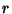, Circumradius, and Areacan be computed directly from the formulas for a general regular Polygon with side lengthand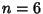sides,(1)(2)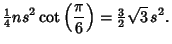(3)

Therefore, for a regular hexagon,(4)

so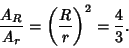(5)

A Plane Perpendicular to a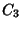axis of a Cube, Dodecahedron, or Icosahedron cuts the solid in a regular Hexagonal Cross-Section (Holden 1991, pp. 22-23 and 27). For the Cube, the Plane passes through the Midpoints of opposite sides (Steinhaus 1983, p. 170; Cundy and Rollett 1989, p. 157; Holden 1991, pp. 22-23). Since there are four such axes for the Cube and Octahedron, there are four possible hexagonal cross-sections.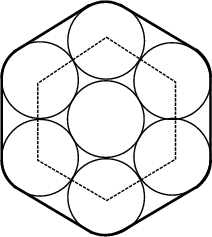Take seven Circles and close-pack them together in a hexagonal arrangement. The Perimeter obtained by wrapping a band around the Circle then consists of six straight segments of length(whereis the Diameter) and 6 arcs with total length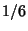of a Circle. The Perimeter is therefore(6)

See also Cube, Cyclic Hexagon, Dissection, Dodecahedron, Graham's Biggest Little Hexagon, Hexagon Polyiamond, Hexagram, Magic Hexagon, Octahedron, Pappus's Hexagon Theorem, Pascal's Theorem, Talisman Hexagon

References

Cundy, H. and Rollett, A. Hexagonal Section of a Cube.'' §3.15.1 in Mathematical Models, 3rd ed. Stradbroke, England: Tarquin Pub., p. 157, 1989.

Dixon, R. Mathographics. New York: Dover, p. 16, 1991.

Holden, A. Shapes, Space, and Symmetry. New York: Dover, 1991.

Pappas, T. Hexagons in Nature.'' The Joy of Mathematics. San Carlos, CA: Wide World Publ./Tetra, pp. 74-75, 1989.

Steinhaus, H. Mathematical Snapshots, 3rd American ed. New York: Oxford University Press, 1983.